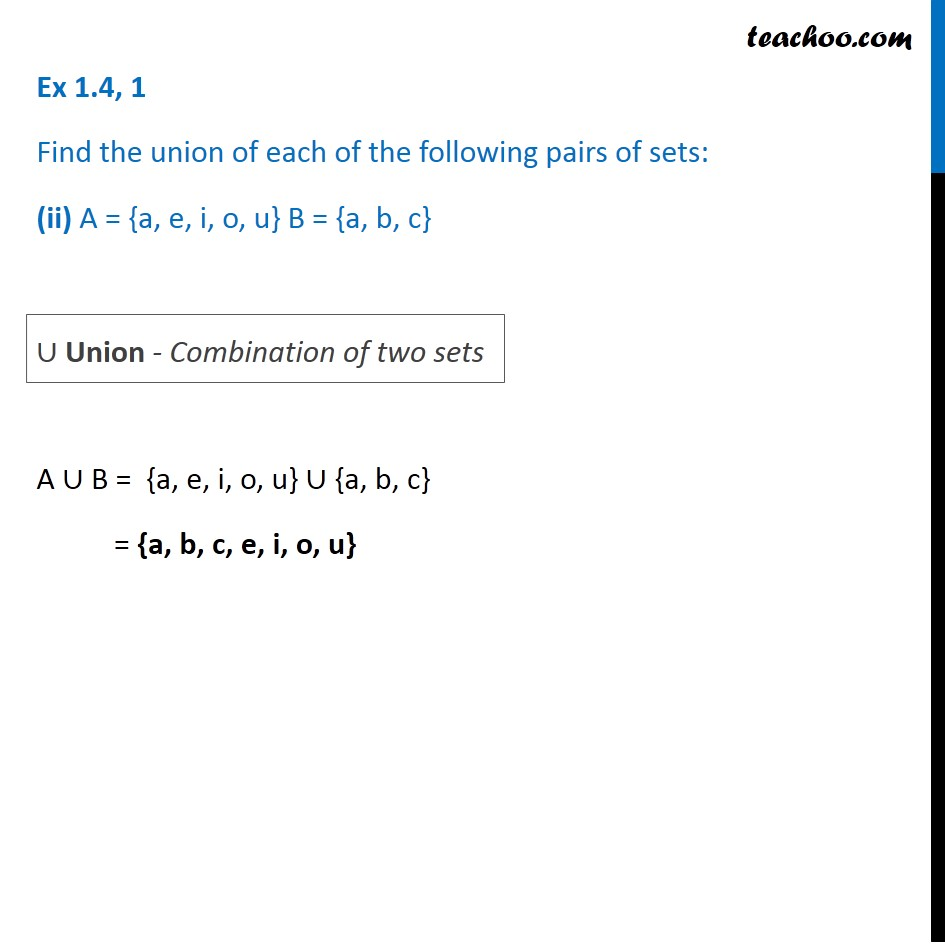1. Chapter 1 Class 11 Sets (Term 1)
2. Serial order wise
3. Ex 1.4

Transcript

Ex 1.4, 1 Find the union of each of the following pairs of sets: (ii) A = {a, e, i, o, u} B = {a, b, c} A ∪ B = {a, e, i, o, u} ∪ {a, b, c} = {a, b, c, e, i, o, u} ∪ Union - Combination of two sets